# Units in Thermochemical Calculations

Most introductory chemistry texts include an example of a calculation for the Gibbs energy change of a chemical reaction not unlike the following:

For the reaction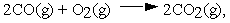calculate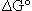at 298 K from the tabulated standard Gibbs energies of formation.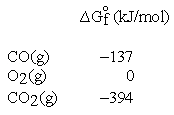These and other standard formation parameters refer to the formation of the compound of interest in its standard state from the elements in their standard states. For example: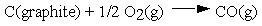.

Thus, the standard Gibbs energy of formation for CO is -137 kJ per mole of CO.

The procedure outlined for the calculation is: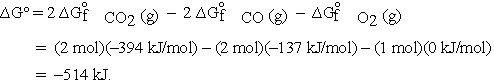Several pages later, conveniently separated from the calculation of, the student is asked to calculate the equilibrium constant for the reaction from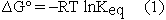.

However, at this point it is generally, but not always, recognized that there is a problem.1 Let us return to the original calculation of. Here we saw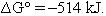. Now we use eqn (1):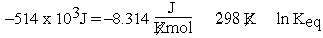and notice that the units don't match. Different texts treat this dilemma in different ways. Some point out that the units forshould be J/mol but don't say why, some change the units of R, and some ignore the discrepancy.

The origin of the difficulty is that the symbolas used in equation (1) refers to an intensive quantity not an extensive quantity; therefore the units ofmust be energy/mol. In part the problem is that the stoichiometric coefficients of the equation are not recognized as being unitless.2 In fact, all thermodynamic quantities calculated for chemical reactions, enthalpy, Gibbs energy, and entropy must have units that show the intensive property of each of the parameters; i.e., units that should be on a per mole basis. If the delta symbol were changed to reflect this intensive character, perhaps some of the misunderstanding could be alleviated, but it is unlikely that a symbol change will ever be effected.

The stoichiometric coefficients can be thought of as representing the number of moles of a species participating in a chemical reaction per mole of reaction. One mole of reaction (molrxn) refers to a reaction unit. In order to define a mole of reaction the chemical equation must be known. In our example the coefficient for CO would become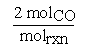where molrxn refers to the reaction as written.

Similarly, for O2 the coefficient would be written2,3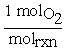. For a chemical reaction, the change in moles for each species is tied to molrxn by the stoichiometric coefficient. So in our reaction, if two moles of CO react with one mole of O2 to produce two moles of CO2,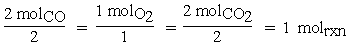then one mole of reaction is said to have occurred.

The calculation now becomes: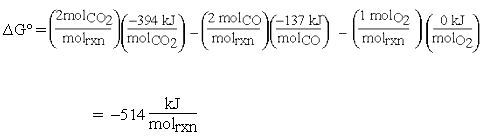The units for equation (1) are now consistent: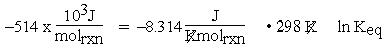Note that here molrxn may be substituted for mol in the units of R because the molrxn connects the amount of reaction to the chemical equation through the coefficients. Thus, molrxn is the same for all species appearing in the chemical equation.

As mentioned above, other reaction thermodynamic parameters must also be intensive; i.e., have units using a per mole basis. If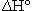is calculated for the previous reaction from tabulated enthalpy of formation data at 298 K: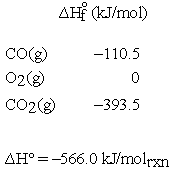If the units forare given as kJ rather than kJ/molrxn, and we wantfor the reaction of 0.10 mol of CO, the procedure is rather strained. First, we need to recognize that the -566.0 kJ actually applies to the consumption of two moles of CO. That seems to imply that we need to divide kJ by moles so that now we have a unit on a per mole basis. We can then multiply by 0.10 moles so that the mole units cancel and the answer is obtained in kJ. In some cases students are told to divide by two but this implies that the coefficient is unitless, whereas the calculation to obtain the units of kJ assigned the mole unit to the coefficients.for the reaction of 0.10 mol of CO can be calculated in a much more straightforward way by using the previous unit analysis.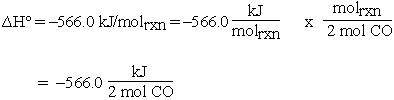Then for 0.10 mol CO:If 0.10 mol CO reacts, 0.050 mol of O2 must react and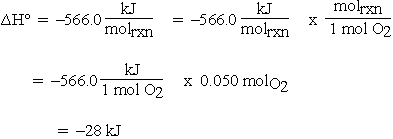DeKock4 has also pointed out that the units in the calculation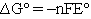are frequently misinterpreted. Here, as with stoichiometric coefficients, n should be unitless and is more clearly written as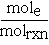, where mole is the number of moles of electrons, thenhas units of kJ/molrxn .

There have been several suggestions1,2,4 on what symbol should be used to designate changes in thermodynamic parameters for reactions. A change that seems to be becoming more commonly accepted is to distinguish between the extensive propertyand the enthalpy change accompanying a chemical reaction by writing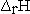for the reaction enthalpy. The reaction Gibbs energy would be written as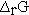. Perhaps the simplest procedure would be to recognize that reaction parameters have units on a per mole basis and that the coefficients are unitless.4

### References

1 Spencer, J.N. Journal of Chemical Education 51 (1974): 577-579.
2 Craig, N.C. Journal of Chemical Education 64 (1987): 668-669.
3 Spencer, J.N., G.M. Bodner, and L.H. Rickard. Chemistry: Structure and Dynamics. 2nd ed. New York: John Wiley & Sons, Inc. (2003): 268-270.
4 DeKock, R.L. Journal of Chemical Education 73 (1996): 955-956.

James N. Spencer is the William G. and Elizabeth R. Simeral Professor of Chemistry at Franklin & Marshall College. He is co-author of a general chemistry text and has written more than 100 articles on research and education. He was chair of the American Chemical Society Task Force on the General Chemistry Curriculum, and a member of the Wisconsin NSF New Traditions Project on teaching. He has been honored with the 1987 Chemical Manufacturer's Association National Teaching Award, the Lindback Teaching Award, the Dewey Award for Research, the 1999 American Chemical Society National Award for Research at an Undergraduate Institution, and the 2000 Mid-Atlantic ACS Emmet Reid Teaching Award. He is currently a member of the AP Chemistry Development Committee.

### Acknowledgment:

The author wishes to thank Haralambos Tzinis for his thoughtful and critical reading of this manuscript. His insightful comments helped make a difficult topic easier to comprehend.

### Authored by

James N. Spencer
Franklin & Marshall College
Lancaster, Pennsylvania